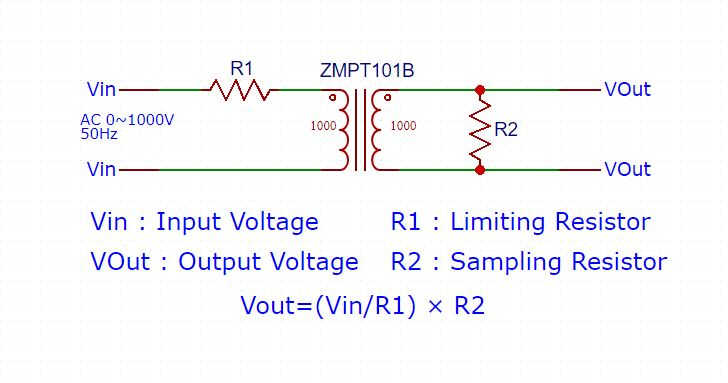# ZMPT101B

ZMPT101B AC Voltage Sensor is the best for the purpose of the DIY project, where we need to measure the accurate AC voltage with the voltage transformer. This is an ideal choice to measure the AC voltage using Arduino/ESP8266/Raspberry Pi like an open source platform. In many electrical projects, engineer directly deals with measurements with few basic requirements like.

• High galvanic isolation
• Wide Range
• High accuracy
• Good Consistency

ZMPT 101B is a high-precision voltage Transformer. This module makes it easy to monitor AC mains voltage up to 1000 volts. If you want smaller version of this module check ZMPT107.

## Applications of Small Size Voltage transformer

• Metering (electrical energy meters)
• AC Voltage measurement
• Ground fault detection
• Household electrical equipment
• Industrial apparatuses
• Electrical testing equipment and relay protection

## Features of ZMPT 101B Micro Precision Voltage Transformers

• low price
• Small size (49.5mm x 19.4mm) and lightweight
• Easy PCB mounting
• Good consistency
• widely acclaimed
SpecificationsDiscriptions
Rated input current2mA
Rated output current2mA
Linear range0~1000V 0~10mA
Isolation withstand voltage4000V
Turns Ratio1000:1000
Measurement accuracy Class0.2
Linearity0.1%
Rated Burdon<=200Ohm
Operating Frequency50-50Hz
DC coil Resistance110 @ 20 deg Cel

## Know More About micro Voltage Transformer ZMPT101B

ZMPT101B is a high-precision voltage Transformer. This module makes it easy to monitor AC mains voltage up to 1000 volts. A tiny little thing the size of a bouillon cube. Holds up to 4kV per breakdown voltage, the ratio of turns is 1: 1, but this is a current transformer of 2mA: 2mA. We feed it a current and remove the current. The input current is simply set by the resistor in series R1, and we use a sampling resistor R2 in parallel to get the output voltage.

## ZMPT101B schematic DiagramNow we get 5 volts, but here an ambush. This voltage is varied from -5 to +5. And we need measurements of 0 … 5 volts. What to do? Straighten.

See in next section.

Note: we use this same calculation for
AC Power Measurement Using Arduino project, where we use ZMPT107 | Best Low Cost AC Voltage Transformer

## ZMPT 101B Transformer Calculation

R1 is chosen so that the current through the winding does not exceed 2mA, it holds a maximum of 10mA, but after 2mA linearity is lost and the output will be clear that.

### Step 1: Determination of maximum output RMS voltage

VOutmax is decided by the ADC peak voltage in the sampling loop of Microcontroller.

VOutmax=(Peak Voltage)/(√2)

For example
As for ± 5V ADC, the maximum RMS voltage of the transformer:

VOutmax=(Peak Voltage)/(√2)=5V/(√2)=3.53V

VOutmax=(Peak Voltage)/(2√2)

For example
As for 0-3.3V ADC, the maximum RMS voltage of the transformer:

VOutmax=(Peak Voltage)/(2√2)=3.3V/(2√2)=1.16V

### Step 2: Determination of input current-limiting resistor R1

Current-limiting resistor

R1=Vin/I

Where
Vin : Rated input voltage
I ： Rated operating current （ when Coil resistance is compared with current-limiting resistor R1, it can be ignored.）

ZMPT101B usually working at rated current: 1~2mA.
When Rated input voltage ≤ 100V ，Usually choosing the operating current I=2mA; When Rated input voltage ≥ 220V，To reducing the resistor power， usually choosing the operating current 1 mA ≤ I ≤ 2 mA.
for example V=100V，I=2 mA，

R1=Vin/I=100/0.002=50kΩ

for example: V=220V，I=1.1 mA，

R1=Vin/I=220/0.0011=200kΩ

To improve reliability, the current-limiting resistor selected usually is greater than its 4 times the rated power, and generally, uses a high temperature coefficient metal film resistor.

### Step 3: Determination of the sampling resistor R2

R2=VOutmax/I=(VOutmax/Vin)×R1 Ω

for example: Voutmax =3.53V，Vin =100V

R2=VOutmax/I=(VOutmax/Vin)×R1=(3.53/100)×50 kΩ=1.765kΩ

The above formula is also suitable for the two ways of active and passive output.
When selecting the sampling resistor, Resistor should not exceed

R2=VOutmax/I=VOutmax/Vin×R1 Ω

## Interface ZMPT 101 B Module to Arduino

For Diy Electronic measuring AC Output Voltage using Arduino is easy now.

ZMPT101B is a Single Phase Voltage Transformer Module which allows you to directly measure 0-250V AC sine wave using Arduino.

Description:
Single-phase AC active output voltage mutual inductance module equipped with ZMPT101B series of a high-precision voltage transformer and high-precision op-amp current, easy to 250v within the AC power signal acquisition.## Feature of ZMPT101B Module

• Voltage Transformer: Onboard Precision Micro Voltage Transformer
• Operational amplifier circuit: high-precision on-board amplifier circuit, the signal to do the exact sampling and appropriate compensation.
• Input/Output: the module can measure AC voltage within 250V, the corresponding output voltage amplitude can be adjusted by using a potentiometer( Multi-turn trim pot).
• Output signal: the output signal for the sine wave, the waveform of the median (DC component(offset of 2.5V at 5V input supply)
• Supply voltage: 5V
• Operating temperature: 40ºC ~ + 70ºC